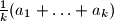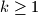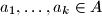### MEMO 2014 ekipno problem 7

Kvaliteta:
Avg: 1.0
Težina:
Avg: 5.0
A finite set of positive integers$A$ is called meanly if for each of its nonempty subsets the arithmetic mean of its elements is also a positive integer. In other words, A is meanly if$\frac{1}{k}(a_1 + \ldots + a_k)$ is an integer whenever$k \geq 1$ and$a_1, \ldots, a_k \in A$ are distinct.

Given a positive integer$n$, determine the least possible sum of the elements of a meanly$n$-element set.
Source: Srednjoeuropska matematička olimpijada 2014, ekipno natjecanje, problem 7